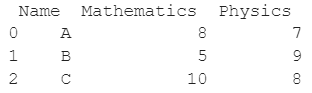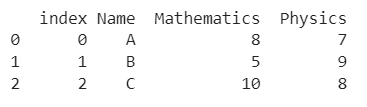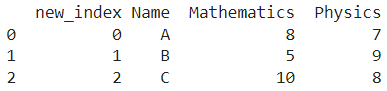# How To Convert The Index Of A Pandas DataFrame Into A Column?

A DataFrame is the primary data structure of the Pandas library and is commonly used for storing and working with tabular data. A common operation that could be performed on such data is getting the row count of an existing DataFrame in order to gauge exactly how many data points we have.

To start working with Pandas, we first need to import it into the Python code:

### Python 3 Code :

``import pandas as pd``

## Running Example

Let us understand this operation with the help of an example. Consider the following DataFrame containing 3 students with names A, B, and C and their corresponding marks (out of 10) for two subjects, Mathematics and Physics.Code snippet for generating the above DataFrame :

### Python 3 Code :

``````import pandas as pd

# Dictionary for our data
data = {'Name' : ['A', 'B', 'C'], 'Mathematics' : [8, 5, 10], 'Physics' : [7, 9, 8]}

# DataFrame for the dictionary
df = pd.DataFrame(data)

# Printing the DataFrame
print(df)``````

Here, data is a dictionary we created to initialize the DataFrame. For this, we use the DataFrame() function of the Pandas library which takes the dictionary as an argument and returns the required DataFrame.

Now let us say that for some reason, we need to convert the index of this DataFrame into a column. The resulting DataFrame would look as follows :

Expected Output :Let us look at different ways of performing this operation :

## Convert The Index Of A Pandas DataFrame Into A Column Using the DataFrame.reset_index() function

In this method, we use the DataFrame.reset_index() function to convert the index of a Pandas DataFrame into a column. This function returns the updated DataFrame.

The returned DataFrame has to be reassigned to the original variable since the update is not in place.

By default, the label of the new column, that is added to the DataFrame, is index.

Let us look at the code for this method and the corresponding output.

### Python 3 Code :

``````import pandas as pd

# Dictionary for our data
data = {'Name' : ['A', 'B', 'C'], 'Mathematics' : [8, 5, 10], 'Physics' : [7, 9, 8]}

# DataFrame for the dictionary
df = pd.DataFrame(data)

# Adding a new column History with a constant value of 7 to the DataFrame df
df = df.reset_index()

# Printing the updated DataFrame
print(df)``````

Output :## Convert The Index Of A Pandas DataFrame Into A Column Using the DataFrame.reset_index() function (with in place update)

In this method, we use the DataFrame.reset_index() function to convert the index of a Pandas DataFrame into a column. This function returns the updated DataFrame.

By default, the label of the new column, that is added to the DataFrame, is index and by specifying the parameter inplace as True, we make the update in place and there is no need to reassign the returned DataFrame to the original one.

Let us look at the code for this method and the corresponding output.

### Python 3 Code :

``````import pandas as pd

# Dictionary for our data
data = {'Name' : ['A', 'B', 'C'], 'Mathematics' : [8, 5, 10], 'Physics' : [7, 9, 8]}

# DataFrame for the dictionary
df = pd.DataFrame(data)

# Adding a new column History with a constant value of 7 to the DataFrame df
df.reset_index(inplace = True)

# Printing the updated DataFrame
print(df)``````

Output :## Convert The Index Of A Pandas DataFrame Into A Column Using the DataFrame.index property

In this method, we use the DataFrame.index property to convert the index of a Pandas DataFrame into a column. We create a new column and specify the desired label, ‘index’ here.

The df[‘index’] is used to refer to this new column. The df.index property returns a Pandas index object containing the labels of the rows of the DataFrame.

Let us look at the code for this method and the corresponding output.

### Python 3 Code :

``````import pandas as pd

# Dictionary for our data
data = {'Name' : ['A', 'B', 'C'], 'Mathematics' : [8, 5, 10], 'Physics' : [7, 9, 8]}

# DataFrame for the dictionary
df = pd.DataFrame(data)

# Adding a new column History with a constant value of 7 to the DataFrame df
df['index'] = df.index

# Printing the updated DataFrame
print(df)``````

Output :## Convert The Index Of A Pandas DataFrame Into A Column Using the DataFrame.insert() function

In this method, we use the DataFrame.insert() function to convert the index of a Pandas DataFrame into a column. This function helps us in performing in place addition of the column so there is no need for reassignment.

The first parameter helps us to specify the position of the new column to be added, set as 0 here.

The second parameter, column, specifies the label of the new column to be added, set as index here.

The third parameter, value, specifies the value contained in the column, set as df.index here. df.index returns a Pandas index object containing the labels of the rows of the DataFrame.

Let us look at the code for this method and the corresponding output.

### Python 3 Code :

``````import pandas as pd

# Dictionary for our data
data = {'Name' : ['A', 'B', 'C'], 'Mathematics' : [8, 5, 10], 'Physics' : [7, 9, 8]}

# DataFrame for the dictionary
df = pd.DataFrame(data)

# Adding a new column History with a constant value of 7 to the DataFrame df
df.insert(0, column = "index", value = df.index)

# Printing the updated DataFrame
print(df)``````

Output :## Convert The Index Of A Pandas DataFrame Into A Column Using the DataFrame.rename_axis() function

In this method, we use the DataFrame.rename_axis() function and DataFrame.reset_index() function to convert the index of a Pandas DataFrame into a column.

This approach is useful when you do not want the new column to have the default label of index but something else, say new_index here.

Let us look at the code for this method and the corresponding output.

### Python 3 Code :

``````import pandas as pd

# Dictionary for our data
data = {'Name' : ['A', 'B', 'C'], 'Mathematics' : [8, 5, 10], 'Physics' : [7, 9, 8]}

# DataFrame for the dictionary
df = pd.DataFrame(data)

# Adding a new column History with a constant value of 7 to the DataFrame df
df = df.rename_axis('new_index').reset_index()

# Printing the updated DataFrame
print(df)``````

Output :## Conclusion

In this topic, we have learned to convert the index of a Pandas DataFrame into a column, following a running example of test scores of students in different subjects, thus giving us an intuition of how this concept could be applied in the real-world situations. Feel free to reach out to info.javaexercise@gmail.com in case of any suggestions.

Trending